x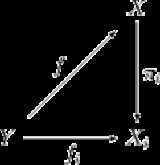Product (category theory)Encyclopedia
In category theory
Category theory
Category theory is an area of study in mathematics that examines in an abstract way the properties of particular mathematical concepts, by formalising them as collections of objects and arrows , where these collections satisfy certain basic conditions...

, the product of two (or more) objects in a category is a notion designed to capture the essence behind constructions in other areas of mathematics such as the cartesian product of sets
Cartesian product
In mathematics, a Cartesian product is a construction to build a new set out of a number of given sets. Each member of the Cartesian product corresponds to the selection of one element each in every one of those sets...

, the direct product of groups
Direct product of groups
In the mathematical field of group theory, the direct product is an operation that takes two groups and and constructs a new group, usually denoted...

, the direct product of rings and the product of topological spaces
Product topology
In topology and related areas of mathematics, a product space is the cartesian product of a family of topological spaces equipped with a natural topology called the product topology...

. Essentially, the product of a family of objects is the "most general" object which admits a morphism to each of the given objects.

## Definition

Let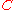be a category with some objects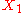and. An object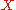is the product ofand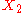, denoted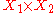, iff it satisfies this universal property
Universal property
In various branches of mathematics, a useful construction is often viewed as the “most efficient solution” to a certain problem. The definition of a universal property uses the language of category theory to make this notion precise and to study it abstractly.This article gives a general treatment...

:
there exist morphisms, called the canonical projections or projection morphisms, such that for every object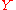and pair of morphismsthere exists a unique morphismsuch that the following diagram commutes
Commutative diagram
In mathematics, and especially in category theory, a commutative diagram is a diagram of objects and morphisms such that all directed paths in the diagram with the same start and endpoints lead to the same result by composition...

: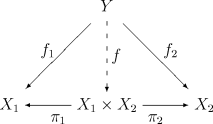The unique morphismis called the product of morphisms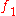and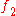and is denoted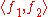.

Above we defined the binary product. Instead of two objects we can take an arbitrary family
Indexed family
In mathematics, an indexed family is a collection of values that are associated with indexes. For example, a family of real numbers, indexed by the integers is a collection of real numbers, where each integer is associated with one of the real numbers....

of objects indexed by some set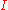. Then we obtain the definition of a product.

An objectis the product of a familyof objects iff there exist morphisms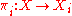, such that for every objectand a-indexed family of morphisms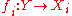there exists a unique morphismsuch that the following diagrams commute for all: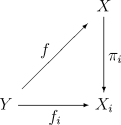The product is denoted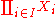; if, then denoted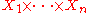and the product of morphisms is denoted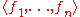.

Alternatively, product may be defined totally by equations, here is an example for binary product:
• Existence of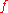is guaranteed by the operation.
• Commutativity of diagrams above are guaranteed by the equality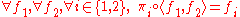.
• Uniqueness ofis guaranteed by the equality.

Also product may be derived from limit
Limit (category theory)
In category theory, a branch of mathematics, the abstract notion of a limit captures the essential properties of universal constructions such as products and inverse limits....

. A family of objects is a diagram
Diagram (category theory)
In category theory, a branch of mathematics, a diagram is the categorical analogue of an indexed family in set theory. The primary difference is that in the categorical setting one has morphisms. An indexed family of sets is a collection of sets, indexed by a fixed set; equivalently, a function...

without morphisms. If we regard our diagram as a functor, it is a functor fromconsidered as a discrete category
Discrete category
In mathematics, especially category theory, a discrete category is a category whose only morphisms are the identity morphisms. It is the simplest kind of category...

. Then the definition of product coincides with the definition of limit,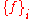being a cone
Cone (category theory)
In category theory, a branch of mathematics, the cone of a functor is an abstract notion used to define the limit of that functor. Cones make other appearances in category theory as well.-Definition:...

and projections being the limit (limiting cone).

As well as limit, product may be defined via universal property. For comparison see Limit#Universal property. Lets unfold that definition for binary product. In our caseis a discrete category with two objects,is simply the product category
Product category
In the mathematical field of category theory, the product of two categories C and D, denoted and called a product category, is a straightforward extension of the concept of the Cartesian product of two sets.-Definition:..., diagonal functor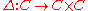assigns to each objectthe ordered pair
Ordered pair
In mathematics, an ordered pair is a pair of mathematical objects. In the ordered pair , the object a is called the first entry, and the object b the second entry of the pair...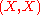and to each morphismthe pair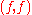. The productinis given by a universal morphism from the functor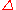to the object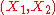in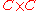. This universal morphism consists of an objectofand a morphismwhich contains projections.

## Examples

• In the category Set (the category of sets
Category of sets
In the mathematical field of category theory, the category of sets, denoted as Set, is the category whose objects are sets. The arrows or morphisms between sets A and B are all functions from A to B...

), the product in the category theoretic sense is the cartesian product
Cartesian product
In mathematics, a Cartesian product is a construction to build a new set out of a number of given sets. Each member of the Cartesian product corresponds to the selection of one element each in every one of those sets...

. Given a family of sets Xi the product is defined as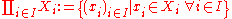with the canonical projectionsGiven any set Y with a family of functionsthe universal arrow f is defined as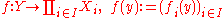• In the category of topological spaces
Category of topological spaces
In mathematics, the category of topological spaces, often denoted Top, is the category whose objects are topological spaces and whose morphisms are continuous maps. This is a category because the composition of two continuous maps is again continuous...

, the product is the space whose underlying set is the cartesian product and which carries the product topology
Product topology
In topology and related areas of mathematics, a product space is the cartesian product of a family of topological spaces equipped with a natural topology called the product topology...

.

• In the category of modules over some ring R, the product is the cartesian product with addition defined componentwise and distributive multiplication.

• In the category of groups
Category of groups
In mathematics, the category Grp has the class of all groups for objects and group homomorphisms for morphisms. As such, it is a concrete category...

, the product is given by the cartesian product with multiplication defined componentwise.

• In the category of algebraic varieties
Algebraic variety
In mathematics, an algebraic variety is the set of solutions of a system of polynomial equations. Algebraic varieties are one of the central objects of study in algebraic geometry...

, the categorical product is given by the Segre embedding
Segre embedding
In mathematics, the Segre embedding is used in projective geometry to consider the cartesian product of two or more projective spaces as a projective variety...

.

• In the category of semi-abelian monoid
Monoid
In abstract algebra, a branch of mathematics, a monoid is an algebraic structure with a single associative binary operation and an identity element. Monoids are studied in semigroup theory as they are naturally semigroups with identity. Monoids occur in several branches of mathematics; for...

s, the categorical product is given by the history monoid
History monoid
In mathematics and computer science, a history monoid is a way of representing the histories of concurrently running computer processes as a collection of strings, each string representing the individual history of a process...

.

• A partially ordered set
Partially ordered set
In mathematics, especially order theory, a partially ordered set formalizes and generalizes the intuitive concept of an ordering, sequencing, or arrangement of the elements of a set. A poset consists of a set together with a binary relation that indicates that, for certain pairs of elements in the...

can be treated as a category, using the order relation as the morphisms. In this case the products and coproduct
Coproduct
In category theory, the coproduct, or categorical sum, is the category-theoretic construction which includes the disjoint union of sets and of topological spaces, the free product of groups, and the direct sum of modules and vector spaces. The coproduct of a family of objects is essentially the...

s correspond to greatest lower bounds (meets
Meet (mathematics)
In mathematics, join and meet are dual binary operations on the elements of a partially ordered set. A join on a set is defined as the supremum with respect to a partial order on the set, provided a supremum exists...

) and least upper bounds (joins).

## Discussion

The product does not necessarily exist. For example, an empty product
Empty product
In mathematics, an empty product, or nullary product, is the result of multiplying no factors. It is equal to the multiplicative identity 1, given that it exists for the multiplication operation in question, just as the empty sum—the result of adding no numbers—is zero, or the additive...

(i.e.is the empty set
Empty set
In mathematics, and more specifically set theory, the empty set is the unique set having no elements; its size or cardinality is zero. Some axiomatic set theories assure that the empty set exists by including an axiom of empty set; in other theories, its existence can be deduced...

) is the same as a terminal object, and some categories, such as the category of infinite groups, do not have a terminal object: given any infinite group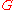there are infinitely many morphisms, socannot be terminal.

Ifis a set such that all products for families indexed withexist, then it is possible to choose the products in a compatible fashion so that the product turns into a functor
Functor
In category theory, a branch of mathematics, a functor is a special type of mapping between categories. Functors can be thought of as homomorphisms between categories, or morphisms when in the category of small categories....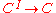. How this functor maps objects is obvious. Mapping of morphisms is subtle, because product of morphisms defined above does not fit. First, consider binary product functor, which is a bifunctor. For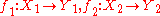we should find a morphism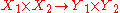. We choose. This operation on morphisms is called cartesian product of morphisms. Second, consider product functor. For families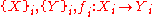we should find a morphism. We choose the product of morphisms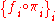.

A category where every finite set of objects has a product is sometimes called a cartesian category
(although some authors use this phrase to mean "a category with all finite limits").

Supposeis a cartesian category, product functors have been chosen as above, and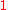denotes the terminal object of. We then have natural isomorphisms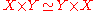These properties are formally similar to those of a commutative monoid
Monoid
In abstract algebra, a branch of mathematics, a monoid is an algebraic structure with a single associative binary operation and an identity element. Monoids are studied in semigroup theory as they are naturally semigroups with identity. Monoids occur in several branches of mathematics; for...

; a category with its finite products constitutes a symmetric monoidal category
Monoidal category
In mathematics, a monoidal category is a category C equipped with a bifunctorwhich is associative, up to a natural isomorphism, and an object I which is both a left and right identity for ⊗, again up to a natural isomorphism...

.

## Distributivity

In a category with finite products and coproducts, there is a canonical morphism X×Y+X×ZX×(Y+Z), where the plus sign here denotes the coproduct
Coproduct
In category theory, the coproduct, or categorical sum, is the category-theoretic construction which includes the disjoint union of sets and of topological spaces, the free product of groups, and the direct sum of modules and vector spaces. The coproduct of a family of objects is essentially the...

. To see this, note that we have various canonical projections and injections which fill out the diagram
The universal property for X×(Y+Z) then guarantees a unique morphism X×Y+X×ZX×(Y+Z). A distributive category
Distributive category
In mathematics, a category is distributive if it has finite products and finite coproducts such that for every choice of objects A,B,C, the canonical map...

is one in which this morphism is actually an isomorphism. Thus in a distributive category, one has the canonical isomorphism• Coproduct
Coproduct
In category theory, the coproduct, or categorical sum, is the category-theoretic construction which includes the disjoint union of sets and of topological spaces, the free product of groups, and the direct sum of modules and vector spaces. The coproduct of a family of objects is essentially the...

– the dual
Dual (category theory)
In category theory, a branch of mathematics, duality is a correspondence between properties of a category C and so-called dual properties of the opposite category Cop...

of the product
• Limit and colimits
Limit (category theory)
In category theory, a branch of mathematics, the abstract notion of a limit captures the essential properties of universal constructions such as products and inverse limits....

• Equalizer
• Inverse limit
Inverse limit
In mathematics, the inverse limit is a construction which allows one to "glue together" several related objects, the precise manner of the gluing process being specified by morphisms between the objects...

• Cartesian closed category
Cartesian closed category
In category theory, a category is cartesian closed if, roughly speaking, any morphism defined on a product of two objects can be naturally identified with a morphism defined on one of the factors. These categories are particularly important in mathematical logic and the theory of programming, in...

• Categorical pullback